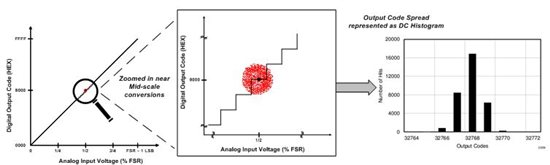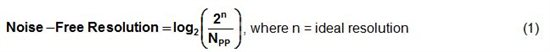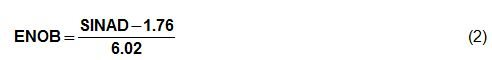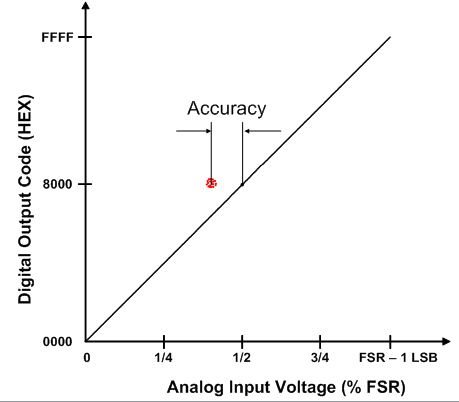This technical article was updated on July 23, 2020.

In talking to system designers using analog-to-digital converters (ADCs), one of the most common questions that I hear is:

The answer to this question lies in the fundamental understanding of the difference between the concept of resolution and accuracy. Despite being two completely different concepts, these two terms are often confused and used interchangeably.

Today’s blog post details the differences between these two concepts. We will dig into the major contributors of ADC inaccuracy in a series of posts.

The resolution of an ADC is defined as the smallest change in the value of an input signal that changes the value of the digital output by one count. For an ideal ADC, the transfer function is a staircase with step width equal to the resolution. However, with higher resolution systems (≥16 bits), the transfer function’s response will have a larger deviation from the ideal response. This is because the noise contributed by the ADC, as well as driver circuitry, can eclipse the resolution of the ADC.

Furthermore, if a DC voltage is applied to the inputs of an ideal ADC and multiple conversions are performed, the digital output should always be the same code (represented by the black dot in Figure 1). In reality, the output codes are distributed over multiple codes (the cluster of red dots seen below), depending on the total system noise (i.e. including the voltage reference and the driver circuitry). The more noise in the system, the wider the cluster of data points is and vice-versa. An example is shown in Figure 1 for mid-scale DC input. This cluster of output points on the ADC transfer function is commonly represented as a DC histogram in ADC datasheets.Figure 1: Illustration of ADC resolution and effective resolution on an ADC transfer curve

The illustration in Figure 1 brings up an interesting question. If the same analog input can result in multiple digital outputs, then does the definition of ADC resolution still hold true? Yes, it does if we only consider the quantization noise of the ADC.  However, when we account for all the noise and distortion in the signal chain, the effective noise-free resolution of the ADC is determined by the output code-spread (NPP), as indicated in equation (1).In typical ADC datasheets, the effective number of bits (ENOB) is specified indirectly by the AC parameter and signal-to-noise and distortion ratio (SINAD), which can be calculated by equation 2:Next, consider if the cluster of output codes (the red dots) in Figure 1 was not centered on the ideal output code and was located somewhere else on the ADC transfer curve away from the black dot (as represented in Figure 2). This distance is an indicator of the data acquisition system’s accuracy. Not only the ADC but also the front-end driving circuit, reference and reference buffers are all contributors to the overall system accuracy.Figure 2: Illustration of accuracy on ADC transfer curve

The important point to be noted, the ADC accuracy and resolution are two different parameters that may not be equal to each other. From a system design perspective, accuracy determines the overall error budgeting of the system, whereas the system software algorithm integrity, control and monitoring capability depend on the resolution.

In my next post, I’ll talk about key factors that determine the “total” accuracy of data acquisition systems.

Related posts on the hub:

• When specifying accuracy, should ADC accuracy be specified in bits, counts or volts (each with a tolerance)? It seems that specifying ADC accuracy in bits is actually specifying a resolution until you add a tolerance. For example, 16-bit ± 2 LSB seems to specify both resolution and accuracy, which both ultimately translate to voltages for a given application.

• In order to define the ADC resolution, the entire transfer function of the ADC has to be accounted for and not a limited section of the transfer function. If you assume a perfectly ideal ADC, then in your example a change in 10mV will not always change the digital output code by 1 count (change from 0.895V to 0.905V will not result in output change), whereas a change in 1V will always change output code by 1 count.

I agree to you that the precision of the ADC is dependent on the offset, gain and non-linearity errors which is explained in more details in the part-II of this series (e2e.ti.com/.../adc-accuracy-part-2-total-unadjusted-error-explained.aspx).

You are right about the definition of SINAD being in dB units. This is how the SINAD is specified in ADC datasheets, as I mentioned in the post above. So, if the SINAD (in dB) as specified in ADC datasheets is used in the formula, then ENOB can be calculated.

In sigma-deltas, the averaging can result in higher resolution but this post is based on Nyquist-sampled ADCs which is the basis for the formula for ENOB mentioned in equation 2. However, the basic conceptual difference between accuracy and resolution is applicable to both nyquist-sampled as well as oversampled ADCs.

• I would like more precise definitions.

So the resolution is not " the smallest change in the value of an input signal that changes the value of the digital output by one count" but (for linear ADCs) the average value achieved by input signal range divided  by number of steps inside this range. An example: on an ideal 4-bit ADC with a range of 16 V the resolution is 1 V, but you can see a change of the value of the digital output by changing the input only from 0.495 V to 0.505 V, a change of only10 mV.

The missing precision of the transfer function of an ADC is dependent on offset, slope, differential and integral nonlinearity, not on noise. You can use this feature by adding noise to the signal to upgrade the resolution of an (low-resolution) ADC!

The definition of ENOB does not contain any dimensions: ENOB is a dimensionless value, ok. If SINAD is treated as dimensionless then a signal of 1 V with a noise&distortion of 10 mV (what is the amplitude of a sum of statistical independent signals like noise and distortion?) would result in a SINAD of 100 resulting in wrong values. Usually  SINAD is given in dB as well as the values 1.76 and 6.02, so it is a logarithmic value: for voltages 20 time the decadic logarithm of signal divided by "noise and distortion" (1 Bel is a factor of 10 for powers).

In a system the achieved resolution is well dependent from software. As example you can see on sigma-delta ADCs that the software algorithms (averaging, filtering) can influence or even define the resolution of the ADC.